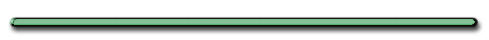Did you know lightning
can go from the ground up?Coulombs Law Notes
Fill In The Blank Notes

F) Coulomb's Law for two fixed point charges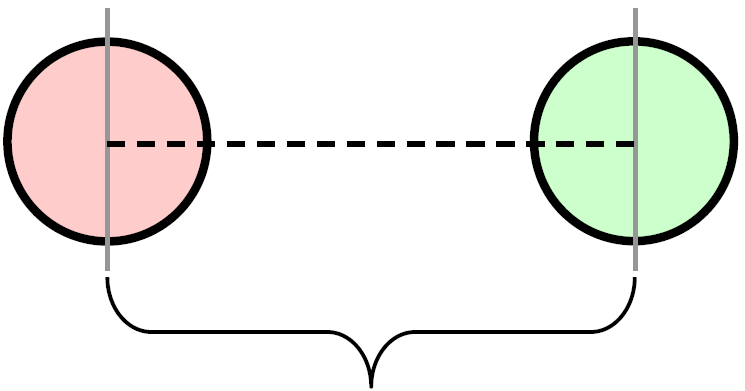r = distance between charges

 F = (N) kq1 q2 r2

F -  mutual force of attraction or repulsion

q - charge of each object

k - electrostatic constant -

8.99 x 109 Nm2/C2
(p. 1 Reference)

AP Physics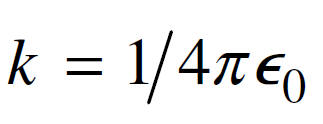AP Physics

Giancoli p. 465) 12

12.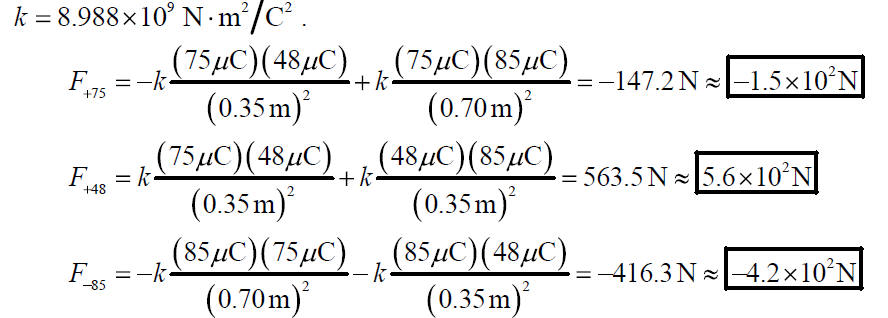Ex) What is the electrical force between 2 very small objects located .50 m apart when the charge on one object is 4.0 x 10-8 C and the charge on the second object is 6.0 x 10-5 C

Ex) What is the electrical force between 2 very small objects located .50 m apart when the charge on one object is 4.0 x 10-8 C and the charge on the second object is 6.0 x 10-5 C

r = .50 meters

q1 = 4.0 x 10-8 C

q2 = 6.0 x 10-5 C

k = 8.99 x 109 Nm2/C2
(p. 1 Reference)

 F = (N) k q1 q2 r2

 Scientific Notation/TI83Plus

More

 F = (8.99 x 109 Nm2/C2) (4.0 x 10-8 C) 6.0 x 10-5 C (.50 m2)

F = 8.6 x 10–2 Newtons

Relationships

 F = (N) k q1 q2 r2

F,r

Inverse Square

Relationship between

F and r?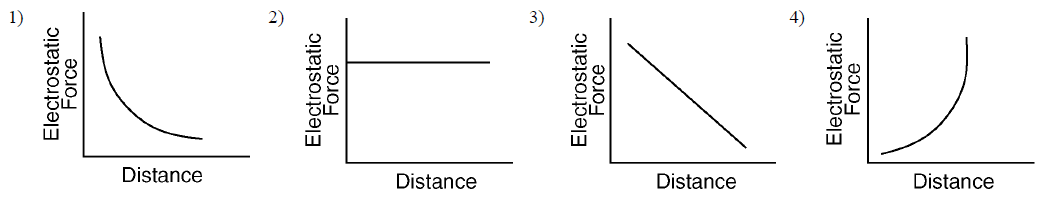How would doubling the distance between the charges change the force between them?

F would be 1/4 as great

F,q?

 F = (N) k q1 q2 r2

Direct

Two charges attract each other with a force of F.  If one charged was doubled and the other charge was tripled, how would that change the attractive force between these charges?

 F = (N) k q1 q2 r2

Force increases 6 timesElectric Field